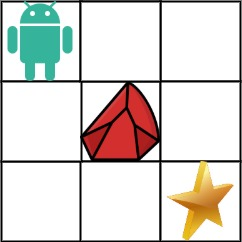``package questionsimport _utils.UseCommentAsDocumentationimport utils.shouldBe/** * A robot is located at the top-left corner of a m x n grid (marked 'Start' in the diagram below). * The robot can only move either down or right at any point in time. * The robot is trying to reach the bottom-right corner of the grid (marked 'Finish' in the diagram below). * Now consider if some obstacles are added to the grids. How many unique paths would there be? * An obstacle and space is marked as 1 and 0 respectively in the grid. * * <img src="https://assets.leetcode.com/uploads/2020/11/04/robot1.jpg" height="200" width="200"/> * * [Source](https://leetcode.com/problems/unique-paths-ii/) */@UseCommentAsDocumentationprivate interface UniquePathsII {    fun uniquePathsWithObstacles(obstacleGrid: Array<IntArray>): Int}private class UniquePathsIINaiveApproach : UniquePathsII {    private enum class Dir { R, D }    private var answer = 0    private lateinit var grid: Array<IntArray>    override fun uniquePathsWithObstacles(obstacleGrid: Array<IntArray>): Int {        if (obstacleGrid[obstacleGrid.lastIndex][obstacleGrid.lastIndex] == 1) {            return 0        }        if (obstacleGrid == 1) {            return 0        }        this.grid = obstacleGrid        val m = obstacleGrid.size        val n = obstacleGrid.size        if (m == 1 && n == 1) return 1        traverse(Dir.R, 0, 0, m - 1, n - 1) // go right        traverse(Dir.D, 0, 0, m - 1, n - 1) // go down        return answer    }    private fun traverse(direction: Dir, posX: Int, posY: Int, rowMaxIndex: Int, colMaxIndex: Int) {        if (direction == Dir.R) {            val newPositionX = posX + 1            if (!canMoveHere(row = posY, col = newPositionX)) {                return            }            if (newPositionX == colMaxIndex && posY == rowMaxIndex) {                answer++            } else {                traverse(Dir.R, newPositionX, posY, rowMaxIndex, colMaxIndex)                traverse(Dir.D, newPositionX, posY, rowMaxIndex, colMaxIndex)            }        } else {            val newPositionY = posY + 1            if (!canMoveHere(row = newPositionY, col = posX)) {                return            }            if (posX == colMaxIndex && newPositionY == rowMaxIndex) {                answer++            } else {                traverse(Dir.R, posX, newPositionY, rowMaxIndex, colMaxIndex)                traverse(Dir.D, posX, newPositionY, rowMaxIndex, colMaxIndex)            }        }    }    private fun canMoveHere(row: Int, col: Int): Boolean {        val cell = this.grid.getOrNull(row)?.getOrNull(col)        if (cell == 0) return true        if (cell == 1 || cell == null) return false        return true    }}private class UniquePathsIINaiveArrayOfArray : UniquePathsII {    override fun uniquePathsWithObstacles(obstacleGrid: Array<IntArray>): Int {        if (obstacleGrid[obstacleGrid.lastIndex][obstacleGrid.lastIndex] == 1) {            return 0        }        if (obstacleGrid == 1) {            return 0        }        val m = obstacleGrid.size        val n = obstacleGrid.size        val dp = Array<IntArray>(m) {            IntArray(n) { 0 }        }        for (row in 0..dp.lastIndex) {            for (col in 0..dp.lastIndex) {                if (obstacleGrid[row][col] == 1) {                    dp[row][col] = 0                } else if (row == 0 && col == 0) {                    dp[row][col] = 1 // only one way to get to (0,0) is moving left                } else if (row == 0) {                    dp[row][col] = dp[row][col - 1] // only way to get to first row is move up                } else if (col == 0) {                    dp[row][col] = dp[row - 1][col] // only way to get to left col is move left                } else {                    dp[row][col] = dp[row - 1][col] + dp[row][col - 1] // two ways                }            }        }        return dp[m - 1][n - 1]    }}fun main() {    val uniquePathCheck: (input: Array<IntArray>, expected: Int) -> Int? = { input, expected ->        UniquePathsIINaiveApproach().uniquePathsWithObstacles(input) shouldBe                UniquePathsIINaiveArrayOfArray().uniquePathsWithObstacles(input) shouldBe expected    }    uniquePathCheck.invoke(arrayOf(intArrayOf(1, 0)), 0)    run {        val input = arrayOf(            intArrayOf(0, 0),            intArrayOf(1, 1),            intArrayOf(0, 0)        )        uniquePathCheck.invoke(input, 0)    }    uniquePathCheck.invoke(        arrayOf(            intArrayOf(0, 0, 0),            intArrayOf(0, 1, 0),            intArrayOf(0, 0, 0)        ),        2    )    uniquePathCheck.invoke(        arrayOf(            intArrayOf(0, 0, 0, 0),            intArrayOf(0, 1, 0, 0),            intArrayOf(0, 0, 0, 0),            intArrayOf(0, 0, 1, 0),            intArrayOf(0, 0, 0, 0),        ),        7    )}``